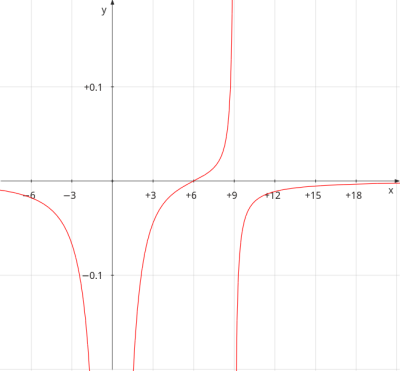# Find a formula for a function f that satisfies the following conditions. \lim_{x \rightarrow...

## Question:

Find a formula for a function f that satisfies the following conditions.

{eq}\lim_{x \rightarrow \pm\infty},f(x)=0\lim_{x \rightarrow 0 },f(x)=-\infty, \\ , \\ \lim_{x \rightarrow 9^{-}},f(x)=\infty , \lim_{x \rightarrow 9^{+}},f(x)=-\infty \\ f(6)=0.\\ f(x)= {/eq}

## Asymptote of a Function:

An asymptote of a function is a line such that its distance to the function approaches to zero as long as the line and the function goes to infinity or to an specific value. The asymptote could be classified as vertical, horizontal or oblique. Horizontal and oblique asymptotes appear when the independent variable goes to infinity and the function approaches to a number. Vertical asymptotes appear when the function goes to infinity while the independent variable approaches to a number.

• A function {eq}\, g(t)\, {/eq} will have a vertical asymptote at {eq}\, w= a\, {/eq} if {eq}\, \displaystyle \lim_{t \rightarrow a}g(t) = \infty {/eq}.
• A function {eq}\, g(t)\, {/eq} will have an horizontal asymptote at {eq}\, t= a\, {/eq} if {eq}\, \displaystyle \lim_{t \rightarrow +\infty}g(t) = a \,\text{ or }\, \displaystyle \lim_{t \rightarrow -\infty}g(t) = a {/eq}.

According to the given limits we know:

• {eq}\displaystyle \lim_{x \rightarrow \pm \infty}f(x) =0 \; \Rightarrow \; {/eq} the function has an horizontal asymptote in {eq}\, y =0 {/eq}.
• {eq}\displaystyle \lim_{x \rightarrow 0}f(x) =-\infty \; \Rightarrow \; {/eq} the function has a vertical asymptote in {eq}\, x=0 {/eq}.
• {eq}\displaystyle \lim_{x \rightarrow 9}f(x) =\infty \; \Rightarrow \; {/eq} the function has a vertical asymptote in {eq}\, x=9 {/eq}.
• {eq}\, f(6)=0 \; \Rightarrow \; {/eq} the function has a root at {eq}\, x=6\, {/eq}.

Consider the function {eq}\, f(x) = \displaystyle \frac{x-6}{x^2(9-x)} \, {/eq}

Notice that the function has a root at {eq}\, x=6\, {/eq} and is undefined at {eq}\, x=0 \; \text{ and }\; x=9 {/eq} and goes to infinity at both values.

The sign of the function is:

{eq}f(x) >0 \; \Rightarrow \; x\in (6,9)\\ f(x) <0 \; \Rightarrow \; x\in (-\infty, 0)\cup (0,6)\cup (9,+\infty) {/eq}

Thus, the signs of each {eq}\, \infty\, {/eq} correspond to the limits given on the exercise.

Finally, the degree of the polynomial in the denominator is higher that the polynomial in the numerator, thus, the function approaches to zero as long as {eq}\, x\rightarrow \infty {/eq}.

The function {eq}\, f(x) = \displaystyle \frac{x-6}{x^2(9-x)} \, {/eq} satisfies all conditions given in the exercise.